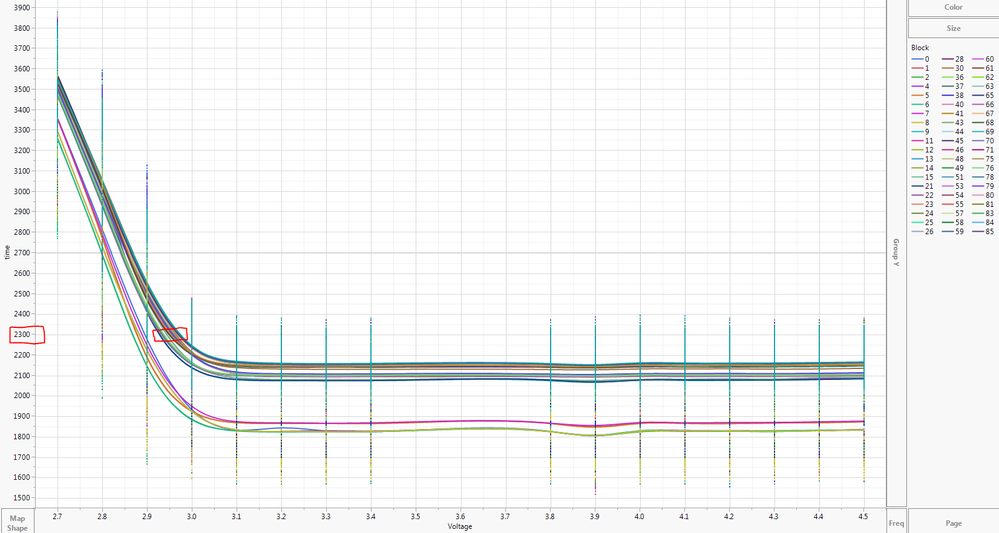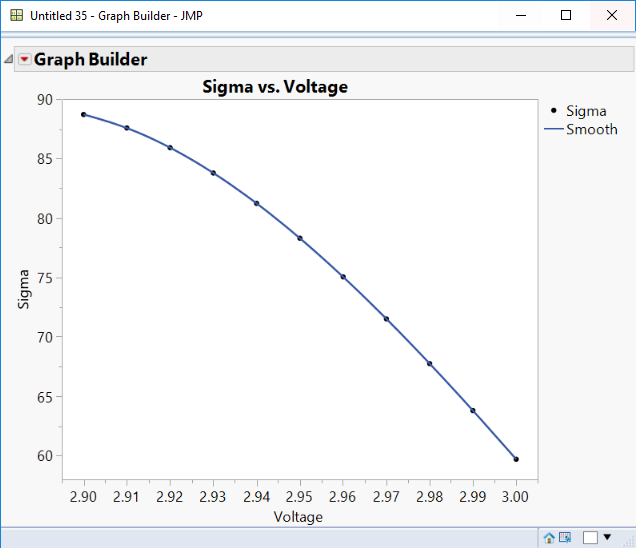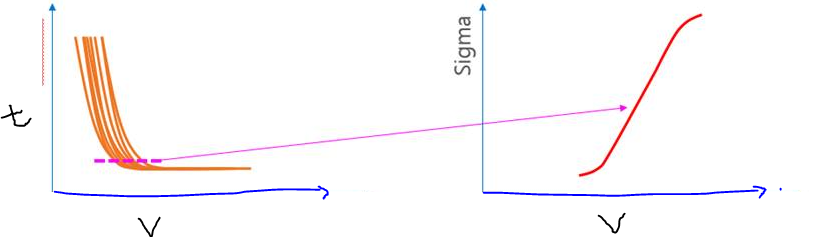Choose Language Hide Translation Bar
Highlighted

## Sigma Plot of smoother curves

Hi,

I want a sigma plot of smoother curves at a time value of 2300 for the upper curves (circled in red, It looks like I have a bi-modal distribution so I just want to focus on the biomodal distrubtion that has more data points). I have no voltage measurements at the circled points (values are between 2.9 and 3.0V). Is there a way to get a sigma plot of these smoother plots at a time of 2300?1 ACCEPTED SOLUTION

Accepted Solutions
Highlighted

## Re: Sigma Plot of smoother curves

Thanks for posting the data.  There's a few splits and stacks to get the data in the right format so we can do the spline fitting, predictions for new values of Voltage, and then compute the standard deviations.  Hope this is clear enough to follow along.  I attached the results of each step.

1. Do a table split on Time, split by Block, Group by Voltage - Result is Table 2 attached
2. On Table 2:
1. Get rid of columns corresponding to block 0 through 8 (these are the lower moded ones you said you didn't want to look at)
2. Do Fit Y by X with Voltage in X, and all the other columns in Y
3. Holding Ctrl, click the red-arrow icon next to "Bivariate Fit of 9 by Voltage" > Flexible > Fit Spline > Other.  Release Ctrl  now and type 0.002 for the smoothness value (this value seemed to work well for me).  Check "Standardize X" as well and press OK.  This should fit smoothers to all of your blocks.
4. Below the graph, there is a red-arrow menu that pertains to the smoothing spline titled "Smoothing Spline Fit, lambda = 0.002 Standardized".  Hold Ctrl again click the red-arrow icon > Save Predicteds.
5. Now you have prediction columns for each block based on the splines and we can predict (interpolate) with new values of Voltage.  Below the last entry in the Voltage column in Table 2, type in 2.9, 2.91, 2.92, ..., 3.  The predicted values will then show up in all of the prediction columns for these voltages.
6. Select the rows you just added (17 throug 27 if you incremented by tenths) and select Tables > Subset and Subset on the selected rows.  This is Table 3.
3. On Table 3:
1. Select all the columns with the original time data for each block and delete them.  You should only have columns "Voltage" and the Spline Predictor columns left.
2. Stack the Spline Predictor Columns (Tables > Stack).  Highlight all of the Spline Predictor columns and click "Stack Columns".  Put "Time Predicted" as the name for the Stacked Data Column.  Press "OK".  Result is Table 4.
4. On Table 4:
1. Create a new column "Sigma".  Add the following formula to the column (you can just paste this in):
``Col Std Dev( :Time Predicted, :Voltage )``
2. You now have standard deviations for each voltage value, so we can plot the results.  Sigma plot looks like so:-- Cameron Willden
11 REPLIES 11
Highlighted

## Re: Sigma Plot of smoother curves

What's a sigma plot?  The Google machine just returns a bunch of information about some overpriced graphing software.  I'm guessing that's not what you meant.

-- Cameron Willden
Highlighted

## Re: Sigma Plot of smoother curves

Here is sigma plot I am trying to create. Yeah I also noticed google giving a bunch of links to buy some softwareHighlighted

## Re: Sigma Plot of smoother curves

Ok thanks for the illustration. That helps, but I'm still a bit confused.  Is sigma denoting standard deviation here?  If so, is it of voltage at a given time point across blocks, or time at a given voltage across blocks?  Neither of those actually make sense to me if you are just interested in Time = 2300 since you would just get a single point to plot.  If we can get on the same page on what is the Y-axis is measuring, then I think it will be easy enough to get the plot you're after.

-- Cameron Willden
Highlighted

## Re: Sigma Plot of smoother curves

Sorry for the confusion. Sigma plot is denoting standard deviation. I want voltage at a given time of 2300 across the blocks. I want to emphasize that I want the sigma plot on the curves that have been smoothed between 2.9 and 3.0V and not on actual data points (I don't have any voltage points between 2.9 and 3.0V). So in other words it is a sigma plot on teh interpolated values.

Highlighted

## Re: Sigma Plot of smoother curves

So, x-axis is Voltage with min at 2.9V and max at 3.0V, and y-axis is standard deviation of time predictions (interpolated from smoothers) at a given voltage.  Right?

If that's the case, you'll probably need to re-create those smoothing splines in Fit Y by X for each block and save the predicted values to the table so you can get those predictions.  If you have each block as a separate column, you can add a few more rows to the get the predicted time at each voltage value you want.  Then, you could compute the standard deviation of the predicted values for each row to get the sigma values for your plot.  If you could post your data table, I could show you what I mean.

-- Cameron Willden
Highlighted

## Re: Sigma Plot of smoother curves

Yes this is exactly what I want. Attaching data table.

Highlighted

## Re: Sigma Plot of smoother curves

Thanks for posting the data.  There's a few splits and stacks to get the data in the right format so we can do the spline fitting, predictions for new values of Voltage, and then compute the standard deviations.  Hope this is clear enough to follow along.  I attached the results of each step.

1. Do a table split on Time, split by Block, Group by Voltage - Result is Table 2 attached
2. On Table 2:
1. Get rid of columns corresponding to block 0 through 8 (these are the lower moded ones you said you didn't want to look at)
2. Do Fit Y by X with Voltage in X, and all the other columns in Y
3. Holding Ctrl, click the red-arrow icon next to "Bivariate Fit of 9 by Voltage" > Flexible > Fit Spline > Other.  Release Ctrl  now and type 0.002 for the smoothness value (this value seemed to work well for me).  Check "Standardize X" as well and press OK.  This should fit smoothers to all of your blocks.
4. Below the graph, there is a red-arrow menu that pertains to the smoothing spline titled "Smoothing Spline Fit, lambda = 0.002 Standardized".  Hold Ctrl again click the red-arrow icon > Save Predicteds.
5. Now you have prediction columns for each block based on the splines and we can predict (interpolate) with new values of Voltage.  Below the last entry in the Voltage column in Table 2, type in 2.9, 2.91, 2.92, ..., 3.  The predicted values will then show up in all of the prediction columns for these voltages.
6. Select the rows you just added (17 throug 27 if you incremented by tenths) and select Tables > Subset and Subset on the selected rows.  This is Table 3.
3. On Table 3:
1. Select all the columns with the original time data for each block and delete them.  You should only have columns "Voltage" and the Spline Predictor columns left.
2. Stack the Spline Predictor Columns (Tables > Stack).  Highlight all of the Spline Predictor columns and click "Stack Columns".  Put "Time Predicted" as the name for the Stacked Data Column.  Press "OK".  Result is Table 4.
4. On Table 4:
1. Create a new column "Sigma".  Add the following formula to the column (you can just paste this in):
``Col Std Dev( :Time Predicted, :Voltage )``
2. You now have standard deviations for each voltage value, so we can plot the results.  Sigma plot looks like so:-- Cameron Willden
Highlighted

## Re: Sigma Plot of smoother curves

So this is close but not exactly what I need. In table 2 you give voltage inputs from 2.9 to 3.0V and then from this you can get the corresponding predicted time for every block. What I want is the other way around I want to give a time of 2300 and then see what voltage each block will give me. So in table 2 if I could instead give it a time of 2300 and then it would predict what voltage that corresponds to. Sorry for confusion about this :(

I have done the following and got it to work but I have a lot of data and it is not feasible to do this task in the steps below:

1. Plot of time vs voltage plot and grouped my data by block (like the first image). Then I did fit polynomial --> 6 and then I did a save predicteds to have this now in my data table: block0_predict
2. Going back to the data table I have a new column called predicted time. Looking at the predicteds formula for this column I copied it into wolframalpha.com website and set it to solve for x variable (voltage), with y variable (time) set to 2300. From this I got 1 real value and a bunch of imaginary values. Then I took this real value and put it into the last row to approximatly get the time of 2300 (please see last row entry). I assume JMP should know how to solve for voltage for a given time across all the blocks, rather then having me copy the equation generated and have it solved in wolframalpha. I assume JMP can also solve such equations but I just don't know how...
Highlighted

## Re: Sigma Plot of smoother curves

I see no feedback on this is it fair to say that this cannot be done in JMP?

Article Labels

There are no labels assigned to this post.